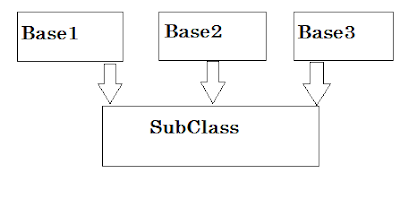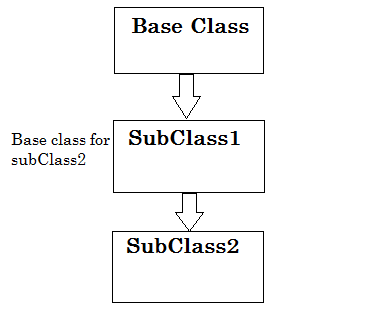Inheritance inheritance is a another feature of oops where one class shares the properties and functions of another class.

For example class B is using the data of class A. Class A is known as base or parent class, and class which is known as derived or subclass. Inheritance use extends keyword to join classes.Introduction of Inheritance. ByTechAchievers.com
``````
class ````A```` {

int x ;

int y;

````void displaySum() {````

x = 3;

y = 4;

System.out.println(" Sum is "+ x+y);

````}````

}

class ````B`` extends ``A```` {

````void displayMultiple() {````

x = 5;

y = 6;

System.out.println(" multiplication is "+ x*y);

````}````

}

``````

Access Modifiers –

In inheritance access modifiers provides hiding mechanism that we can control accessibility of object's data, programmer can decide which property or function will be accessible by other object.

1.  Default – It can be accessible from same package or group of objects only. Its default modifier. It doesn’t need to specify by any keyword.

2.  Public – It can be accessible from anywhere.

3.  Private It cannot be accessible from anywhere, it has access of its specifies object.

4.  Protected – It lies in between default and private, that is can be accessible from same package or group of objects but in case of Inheritance or parent and child relationship objects, only child can access parent data members.

``````
class ````Num ````{

public Integer a;

private Integer b;

protected Integer c;

}

class ````Addition ``extends ``Num ````{

````public void sum() {````

a=2; //can be accessible

b=3; //cannot be accessible

c=5; //c can be accessible

````}````

}
``````
Note – extends keyword use to inherit the class in java.

Types of Inheritance –

Single Inheritance
Single Inheritance is the basic or 1st level of relationship between classes. When one class inheriting features of another class or in very simple way one base class has only one derived class.Single Inheritance. ByTechAchievers.com

Practical Example in Java

``````

class ````Rectangle ````{

public Integer length;

public Integer width;

}

class ````Calculate ``extends ``Rectangle ````{

``````public Integer getArea() {
``````
length=20;

width=30;

return length*width;

`````` }
``````
}

public class ````mainClass ````{

``````public static void main (String args[]) {
``````
Calculate rectangle = new Calculate();

System.out.println(“Rectangle Area is ”+rectangle.getArea());

```` }````

}

``````

Multiple Inheritance –

Multiple Inheritance is when one class inheriting features of multiple classes or one subclass inherits multiple base classes.

Multiple Inheritance increases the complexity in program, subclass has to manage unwanted dependencies as well so that some languages like JAVA, and Smalltalk does not support this inheritance. In C++ we use this inheritance.Multiple Inheritance. ByTechAchievers.com

Multiple Inheritance creates ambiguity problem known as Diamond problem for more details on Diamond problem see the interview questions section in home page.

Multilevel Inheritance –

Multilevel Inheritance is when one subclass inheriting features of base class and again subclass becomes the base for another subclass.

For example SubClass1 inherits Base1 and SubClass2 inherits SubClass1, SubClass1 becomes the Base class for subClass2.See in the diagram for more clarity.Multilevel Inheritance. ByTechAchievers.com

Practical Example in Java

``````
class ````Rectangle```` {

public Integer length;

public Integer width;

protected double r;

}

class ````Calculate ``extends ``Rectangle ````{

public double pi=3.14d;

````public Integer getArea() {````

length=20;

width=30;

return length*width;

````}````

}

class ``````Circle extends Calculate {

public double getArea() {

r=21.4d;

return pi*r*r;

}

}

public class mainClass {

public static void main (String args[]) {

Calculate rectangle = new Calculate();

Circle circle = new Circle();

System.out.println(“Rectangle Area is ”+rectangle.getArea());

System.out.println(“Circle Area is ”+ circle.getArea());

}

}

``````

Hierarchical Inheritance –

Hierarchical Inheritance is the when multiple sub classes inheriting features of one base class .For example baseClass1 is inherited by subClass1, subClass2, subClass3.Hierarchical Inheritance. ByTechAchievers.com

Practical Example in Java

``````
class ````Area ````{

public Integer length;

public Integer width;

protected double r;

protected Integer side;

}

class ````Rectangle ``extends ``Area ````{

``````public Integer getRectangleArea() {
``````
length=20;

width=30;

return length*width;

````}````

}

class ````Circle ``extends ``Area ````{

private double pi=3.14d;

````public double getCircleArea() {````

r=21.4d;

return pi*r*r;

````}````

}

class ````Square ``extends ``Area ````{

````public double getSquareArea() {````

side=4;

return side*side;

````}````

}

public class ````mainClass ````{

```` public static void main (String args[]) {````

Rectangle rectangle = new Rectangle();

Circle circle = new Circle();

Square square = new Square();

System.out.println(“Rectangle Area is ”+rectangle.getRectangleArea());

System.out.println(“Circle Area is ”+ circle.getCircleArea());

System.out.println(“Square Area is ”+ square.getSquareArea());

```` }````

}
``````

Hybrid Inheritance –

Hybrid Inheritance is the combination of multiple and multilevel inheritance where one sub class inherits multiple base classes and these sub classes are again inherited by other subclass.
In C++ we can achieve it but Java does not support multiple inheritance, Java handle this structure using interfaces will discuss it later.Hybrid Inheritance. ByTechAchievers.com